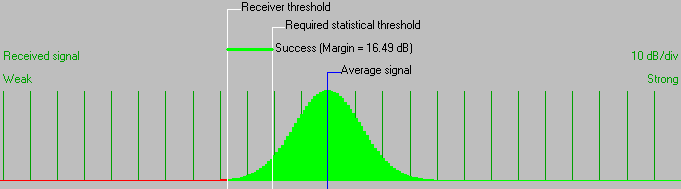• Distance between 03-Roan Mtn NC/TN and 04-Comers Rock, VA is 109.8 km (68.3 miles)
• True North Azimuth = 47.95°, Magnetic North Azimuth = 54.67°, Elevation angle = -0.9826°
• Terrain elevation variation is 1148.2 m
• Propagation mode is line-of-sight, minimum clearance 0.2F1 at 0.5km
• Average frequency is 145.000 MHz
• Free Space = 116.4 dB, Obstruction = 0.0 dB, Urban = 1.0 dB, Forest = 1.0 dB, Statistics = 20.3 dB
• Total propagation loss is 138.7 dB (interference mode (optimistic))
• System gain from 03-Roan Mtn NC/TN to 04-Comers Rock, VA is 155.2 dB
• System gain from 04-Comers Rock, VA to 03-Roan Mtn NC/TN is 155.2 dB
• Worst reception is 16.5 dB over the required signal to meet 95.000% of situations